# Test: Clocks- 2

## 10 Questions MCQ Test UPSC Prelims Paper 2 CSAT - Quant, Verbal & Decision Making | Test: Clocks- 2

Description
Attempt Test: Clocks- 2 | 10 questions in 10 minutes | Mock test for UPSC preparation | Free important questions MCQ to study UPSC Prelims Paper 2 CSAT - Quant, Verbal & Decision Making for UPSC Exam | Download free PDF with solutions
QUESTION: 1

### An accurate clock shows 8 o'clock in the morning. Through how may degrees will the hour hand rotate when the clock shows 2 o'clock in the afternoon?

Solution:

We know that angle traced by hour hand in 12 hrs = 360°

From 8 to 2, there are 6 hours.

Angle traced by the hour hand in 6 hours = 6x(360/12)=180o

QUESTION: 2

### A clock is started at noon. By 10 minutes past 5, the hour hand has turned through

Solution:

We know that angle traced by hour hand in 12 hrs = 360°

Time duration from noon to 10 minutes past 5
= 5 hours 10 minutes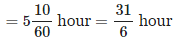Hence the angle traced by hour hand from noon to 10 minutes past 5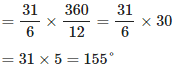QUESTION: 3

### At 3:40, the hour hand and the minute hand of a clock form an angle of

Solution:

Angle between hands of a clock

When the minute hand is behind the hour hand, the angle between the two hands at M minutes past H 'o clock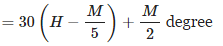When the minute hand is ahead of the hour hand, the angle between the two hands at M minutes past H 'o clock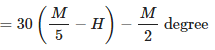QUESTION: 4

The angle between the minute hand and the hour hand of a clock when the time is 8.30, is

Solution: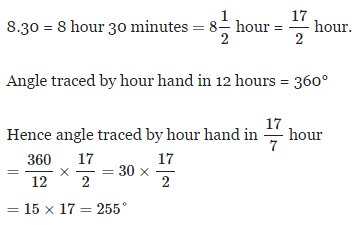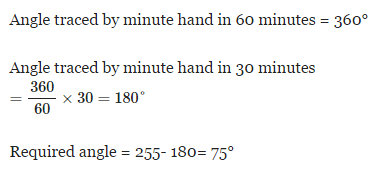QUESTION: 5

How many times will the hands of a clock coincide in a day?

Solution:

The hands of a clock coincide 11 times in every 12 hours (Between 11 and 1, they coincide only once, at 12 o'clock).

12:00 am
1:05 am
2:11 am
3:16 am
4:22 am
5:27 am
6:33 am
7:38 am
8:44 am
9:49 am
10:55 am
12:00 pm
1:05 pm
2:11 pm
3:16 pm
4:22 pm
5:27 pm
6:33 pm
7:38 pm
8:44 pm
9:49 pm
10:55 pm

Hence the hands coincide 22 times in a day.

This is already given as a formula and it's is better to learn the answer by heart as 22 which can save time in competitive exams.(However you should know the theory behind).

QUESTION: 6

At what time between 4 and 5 o'clock will the hands of a watch point in opposite directions?

Solution:

The two hands of a clock will be in the same straight line but not together between HHand (H+1)(H+1) o' clock at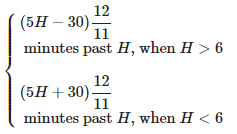Here H = 4. Hands of the watch will point in opposite directions at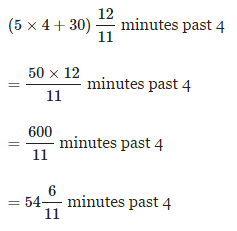QUESTION: 7

A clock is set at 5 am. If the clock loses 16 minutes in 24 hours, what will be the true time when the clock indicates 10 pm on 4th day?

Solution:

Time from 5 am to 10 pm on the 4th day
= 3 days 17 hours
= 3 × 24 + 17
= 89 hours.

Given that clock loses 16 minutes in 24 hours.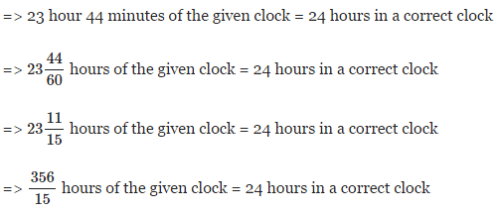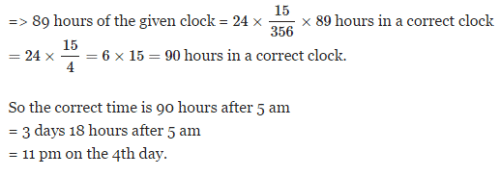QUESTION: 8

The minute hand of a clock overtakes the hour hand at intervals of 65 minutes. How much a day does the clock gain or loss?

Solution:

If the minute hand of a clock overtakes the hour hand at intervals of MM minutes of correct time, the clock gains or loses in a day by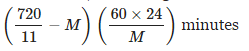Here M = 65. The clock gains or losses in a day by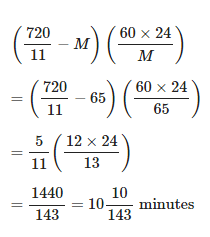QUESTION: 9

Find the time between 4 and 5'o clock, when the two hands of a clock are 4 minutes apart?

Solution:

At 4 o' clock, the hands are 20 minute spaces apart.

To get the first 4 min spaces between the hands ,the minute hand has to gain 20 - 4 = 16 minute spaces over the hour hand.

To get the second 4 min spaces between the hands ,the minute hand has to gain 20 + 4 = 24 minute spaces over the hour hand.

In 60 minutes, minute hand gains 55 minute spaces over the hour hand.

Time taken for gaining 16 minute spaces by minute hand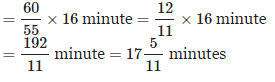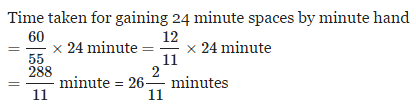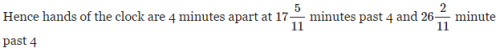QUESTION: 10

What is the angle between the hands at 4.40?

Solution:

Angle between hands of a clock

When the minute hand is behind the hour hand, the angle between the two hands at MM minutes past HH 'o clock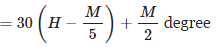When the minute hand is ahead of the hour hand, the angle between the two hands at MM minutes past HH 'o clock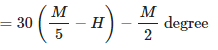Here H = 4, M = 40 the minute hand is ahead of the hour hand. Hence the angle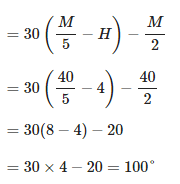Use Code STAYHOME200 and get INR 200 additional OFF Use Coupon Code